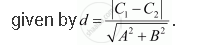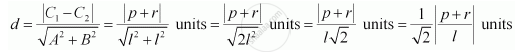# Find the Distance Between Parallel Lines L (X + Y) + P = 0 And L (X + Y) – R = 0 - Mathematics

Find the distance between parallel lines  (y) + = 0 and (y) – = 0

#### Solution

It is known that the distance (d) between parallel lines ABC1 = 0 and ABC2 = 0 isThe given parallel lines are (y) + = 0 and (y) – = 0.

lx ly + = 0 and lx ly – r = 0

Here, A = lB = lC1 = p, and C= –r.

Therefore, the distance between the parallel lines isConcept: Distance of a Point from a Line
Is there an error in this question or solution?

#### APPEARS IN

NCERT Class 11 Mathematics
Chapter 10 Straight Lines
Q 6.2 | Page 227